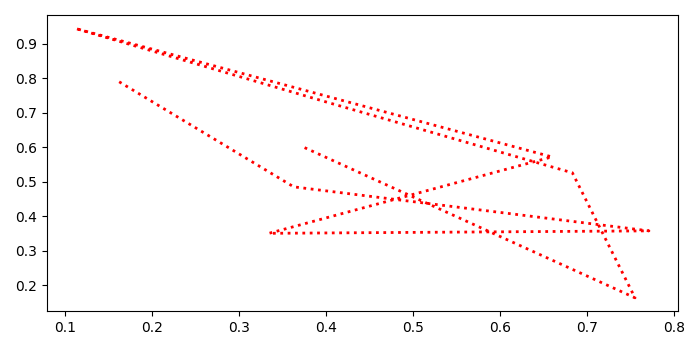# Exporting an svg file from a Matplotlib figure

To export an SVG file from a matplotlib figure, we can take the following steps −

• Set the figure size and adjust the padding between and around the subplots.

• Create a figure and a set of subplots.

• Create random x and y data points using numpy.

• Plot x and y data points using plot() method.

• Save the .svg format file using savefig() method.

## Example

import numpy as np
from matplotlib import pyplot as plt
plt.rcParams["figure.figsize"] = [7.00, 3.50]
plt.rcParams["figure.autolayout"] = True
fig, ax = plt.subplots()
x = np.random.rand(10)
y = np.random.rand(10)
ax.plot(x, y, ls='dotted', linewidth=2, color='red')
plt.savefig("myimg.svg")

## Output

When we execute this code, it will create an SVG file called "myimg.svg" and save it in the current directory.